ChemTalk

# Barometers and Manometers## Core Concepts

In this tutorial, we will discuss barometers and manometers; both are instruments that measure pressure, but they generally measure different types of pressure in different contexts. First, we will explore barometers, different designs, and their uses. Then, we will look at manometers and how to use them with some practice problems.

## Barometers

Barometers traditionally measure atmospheric pressure and therefore can provide forecasts about weather conditions. The most common ones use mercury or water as the liquid inside.

### Mercury Barometers

These barometers are commonly simple designs: a glass tube upside-down in a wide and flat glass bowl, both filled with mercury. Mercury (Hg) is the most common liquid for barometers and manometers because it is nonvolatile (does not evaporate easily) and has a high density. The level of mercury in the glass tube determines the atmospheric pressure. The mercury in the tube will change as its weight balances the force of the atmospheric pressure on the mercury in the bowl (called the reservoir). For example, the mercury in the tube will drop when there is low atmospheric pressure because there is a smaller force on the reservoir. We can read the mercury barometer by the numerical marks on the glass tube.

These barometers can be used to predict the weather; the farther the barometric pressure falls, the stronger the storm. On the contrary, high pressure usually indicates dry, clear skies. Many scientists, including Lucien Vidi, Evangelista Torricelli, Otto von Guericke, conceptualized this.

Fun Fact: “torr,” the unit of pressure, is named after Torricelli! 1 torr is equal to 1mmHg, another unit of pressure.

### Mercury Barometer Practice Problem

Now, let’s try a practice problem using barometric pressure. The weight of the fluid (mercury) creates its own pressure, which we can call hydrostatic pressure. The mercury rises in the tube until its weight matches the atmospheric pressure pressing down on the open surface of the mercury. This means that the hydrostatic pressure equals the atmospheric pressure. Now, we can use this equation below to calculate the hydrostatic (as well as the atmospheric) pressure “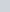“.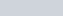In this diagram, the height “” of the mercury is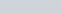. We will convert this to meters, so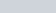, to be able to cancel out units in the equation. The density “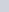” of mercury is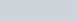, which we can convert to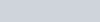. Finally, the gravitational constant “” is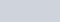.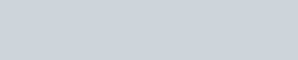Our variablethen equals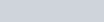, which equals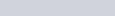or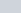. If we convert pascals to atm (another common unit of pressure) using the conversion of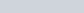=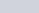, we receivewith significant figures. The atmospheric pressure is therefore. Additionally, this is where the common conversion of=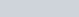comes from, as the height of the mercury in the tube was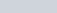. This equation makes finding the pressure easier when other liquids are used, however.

### Water Barometers

Heavy liquids like mercury are desired for the column barometers (like the one in the diagram), so most water barometers have a different design. These barometers are glass and half-filled with water; they have a main body connected to an open and narrow spout. They do not offer quantitative measurements of the atmospheric pressure. Instead, the water level in the spout rises above the water level in the main body when air pressure is low, which provides a relative idea of the atmospheric pressure. This simple design was popular around the 17th century as anyone could use it to predict the weather and storms.

### Aneroid Barometers

Lucien Vidi invented the aneroid barometer in the nineteenth century. This instrument uses a metal box made from an alloy that expands and shrinks as the atmospheric pressure changes. Its circular display shows specific measurements of the air pressure, making these barometers more user-friendly and common than liquid mercury and water barometers.

## Manometers

Manometers are typically used to measure pressure in a closed system of a gaseous matter. A common manometer consists of a U-shaped tube filled with a liquid (usually mercury because of its high density). They are often used in industrial applications to measure pressure in pipes, tanks, and more.

### Closed-Ended Manometers

In a closed-ended manometer, the specific pressure of the gaseous matter equals the difference in height between the mercury on either side of the U-tube. This difference in height is measured in millimeters which becomes millimeters of mercury (mmHg) when written as a gas pressure unit.

For example, in the diagram above, it is a closed-ended manometer with mercury inside. The height difference in mercury between the two sides of the U-tube is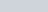, which means that the pressure of the gas is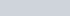(1mmHg is equal to 1 torr, so we can also write this as 30 torr).

### Open-Ended Manometers

In an open-ended manometer, one end of the tube is open, which is where the atmospheric pressure impacts the mercury’s height from. The other end is connected to a system (the gaseous matter where the pressure needs to be measured).

Looking at the heights of the mercury in the U-tube can give us an idea of the gaseous matter’s pressure relative to the atmospheric pressure. If the height of the mercury on the side of the gas is lower than the mercury on the other side, the pressure of the gas is higher than that of the atmosphere. On the other hand, if the height of the mercury on the side of the gas is higher than the mercury on the other side, the pressure of the gas is lower than that of the atmosphere.

If you use an open-ended manometer to measure the pressure of gas, you need to know the exact atmospheric pressure, which we can find with a barometer, as we discussed before.

### Open-Ended Manometer Practice Problem

In the diagram above, it is a open-ended manometer with mercury inside. The problem may give the atmospheric pressure directly or it may require a barometer reading. In this case, the atmospheric pressure is. The height of mercury, because there is an open end, will depend on both the pressure of the gas and the atmospheric pressure. Therefore, the difference in the height of the mercury is equal to the difference in the pressures. The pressure of gas will be equal to the atmospheric pressure plus or minus the difference of the height.

You will ADD the difference in height to the atmospheric pressure if the mercury is higher on the open-ended side. This will also indicate that the gaseous matter’s pressure is lower than the atmospheric pressure.

You will SUBTRACT the difference in height to the atmospheric pressure if the mercury is lower on the open-ended side. This will also indicate that the gaseous matter’s pressure is higher than the atmospheric pressure.

In this diagram above, the difference in height is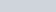, with the mercury being higher on the open-ended side. We will ADD the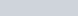to the atmospheric pressure, which the diagram gives as. The gaseous matter’s pressure is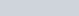## Practice Problems

1. A mercury barometer is set on a level table. The density of the mercury is. The height of the mercury in the glass tube is. What is the atmospheric pressure in pascals?
2. There is an open-ended mercury manometer set on a level table. The mercury height on the left side (the side connected to the gaseous matter) is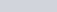. The mercury height on the right side (the open end) is. The atmospheric pressure is. What is the pressure of the gaseous matter in mmHg?

### Practice Problem Solutions

1.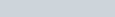2.